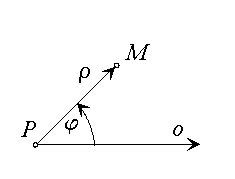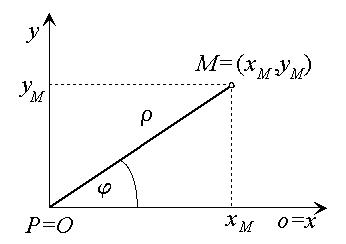# Polar coordinates

Many scientific and technical applications use polar coordinates in the Euclidean space to solve some of the complex geometric problems.

Let P be the chosen fixed point in the plane. The half-line $\stackrel{\to }{o}$ with the start point P and a determined anti-clockwise revolution about P in the plane form the polar coordinate system $\left(P,\phantom{\rule{2mm}{2mm}}\stackrel{\to }{o},\phantom{\rule{2mm}{2mm}}\varphi \right)$. Point P is called the pole - origin of the coordinate system, half-line $\stackrel{\to }{o}$ is the polar axis of the polar coordinate system.Figure 1: Polar coordinates

Choosing the measurement unit, any point M in the plane can be attached an ordered pair of real numbers $M=\left(\rho ,\phantom{\rule{mediummathspace}{0.2em}}\varphi \right)$ with the clear geometric interpretation illustrated in the Figure 1.

1. $\rho =|PM|$ is the distance of the point M from the pole P,
2. $\varphi =|\sphericalangle \left(\stackrel{\to }{o},\stackrel{\to }{PM}\right)|$ is the size of the anti-clockwise oriented angle with vertex in the pole P, which is formed by the polar axis $\stackrel{\to }{o}$ and half-line $\stackrel{\to }{PM}$.

The ordered pair of real numbers $\left(\rho ,\varphi \right)$ form polar coordinates of the point, number $\rho$ is called modul, number $\varphi =\left[0,2\pi \right)$ or $\varphi =\left(-\pi ,\pi \right]$ is called the polar angle.

Let the Cartesian coordinate system (O, x, y) and the polar coordinate system $\left(P,\phantom{\rule{2mm}{2mm}}\stackrel{\to }{o},\phantom{\rule{2mm}{2mm}}\varphi \right)$ be given in the Euclidean plane ${\mathbf{E}}^{2}\left(R\right)$.
These two coordinate systems are called related, if

1. $P=O$, origins of the two coordinate systems coincide
2. Polar axis $\stackrel{\to }{o}$ coincides with the positive part of the coordinate axis x
3. Anti-clockwise orientation in the plane is defined by the revolution of the positive axis x by angle $\varphi =\frac{\pi }{2}$ to the positive axis y

and choosing one the other coordinate system is determined uniqely.Figure 2: Related coordinate systems

If $\left({x}_{M},{y}_{M}\right)$ are Cartesian coordinates and $\left(\rho ,\phantom{\rule{mediummathspace}{0.2em}}\varphi \right)$ are polar coordinates of the point M, their relation can be expressed by equations

 ${x}_{M}=\rho \mathrm{cos}\phantom{\rule{mediummathspace}{0.2em}}\varphi$
 ${y}_{M}=\rho \mathrm{sin}\phantom{\rule{mediummathspace}{0.2em}}\varphi$

and because

 ${x}_{M}^{2}+{y}_{M}^{2}\ne 0$
 $\rho =\sqrt{{x}_{M}^{2}+{y}_{M}^{2}}$

also by equations

 $\mathrm{cos}\phantom{\rule{mediummathspace}{0.2em}}\varphi =\frac{{x}_{M}}{\sqrt{{x}_{M}^{2}+{y}_{M}^{2}}}$
 $\mathrm{sin}\phantom{\rule{mediummathspace}{0.2em}}\varphi =\frac{{y}_{M}}{\sqrt{{x}_{M}^{2}+{y}_{M}^{2}}}$

If $\rho =0$ and thus ${x}_{M}={y}_{M}=0$, the polar angle is not defined by the above equations and the polar coordinates of the point are $P=\left(0,\phantom{\rule{mediummathspace}{0.2em}}\varphi \right)$, where $\varphi \phantom{\rule{2mm}{2mm}}$is an arbitrary number.